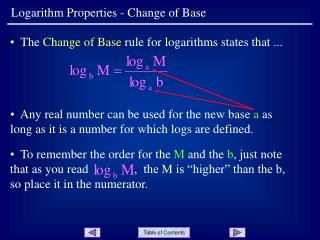# Logarithm Properties - Change of Base - PowerPoint PPT PresentationDownload PresentationLogarithm Properties - Change of Base

Download Presentation## Logarithm Properties - Change of Base

- - - - - - - - - - - - - - - - - - - - - - - - - - - E N D - - - - - - - - - - - - - - - - - - - - - - - - - - -
##### Presentation Transcript

1. To remember the order for the M and the b, just note that as you read , the M is “higher” than the b, so place it in the numerator. Logarithm Properties - Change of Base • The Change of Base rule for logarithms states that ... • Any real number can be used for the new base a as long as it is a number for which logs are defined.

2. Logarithm Properties - Change of Base • Example 1: Slide 2

3. Logarithm Properties - Change of Base • One of the most important uses of the change of base formula is to enter expressions and functions into the calculator. The calculator has keys for both base 10 (LOG) and base e (LN). • Example 2 • Note both values are equal ... Slide 3

4. Logarithm Properties - Change of Base • Example 3: Use a calculator to sketch the graph of the function ... • Use the change of base formula and LN to enter the function into Y1 ... • A ZOOM|ZStandard window yields ... • Note the vertical asymptote at x = 1. Slide 4

5. Logarithm Properties - Change of Base END OF PRESENTATION Click to rerun the slideshow.